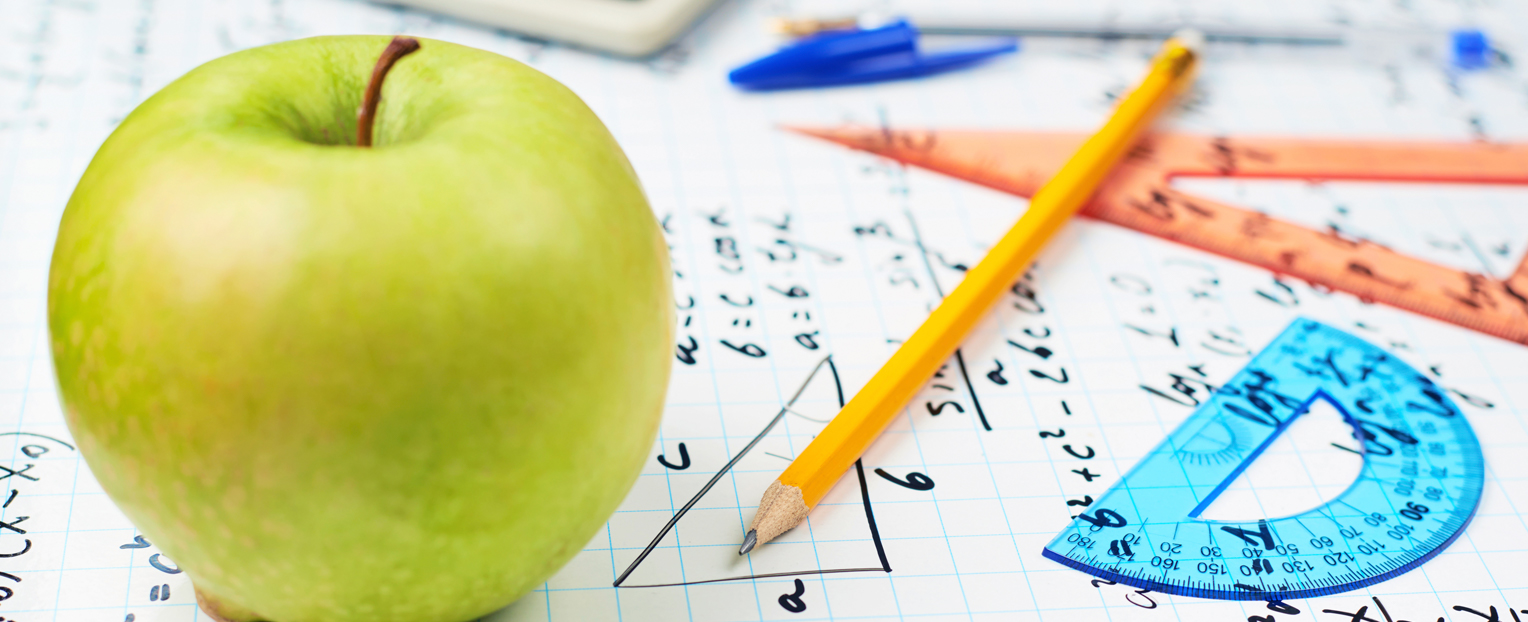From a very young age, children learn and develop using all their senses. As infants they are surrounded by the stimulation of shape, color, lines, numbers, patterns, and textures. By the time they reach preschool, they are engaged in stories and imaginative pursuits that build on these sensory explorations. Thanks to the materials they have explored in their first five years, children already have a strong foundation for mathematics when they start kindergarten.

## Visual Models in the Primary Grades

Current math standards reflect the importance of physical materials in math learning, encouraging Grade K students, for example, to use objects, fingers, drawings, and sounds to count and work out addition and subtraction problems. Counters, hundred charts, songs, Unifix Cubes, measuring devices such as hands and rulers, money, clocks, beads, bread tags, Base Ten blocks, place value flip charts, and rekenreks all have a place in the primary grades math classroom.

Commonly available materials such as dice or number cubes, a deck of cards, real or play money, and dominoes can form the basis of many enjoyable and effective math games.

## Challenges in the Intermediate Grades

As students reach the intermediate grades, however, hands-on math play is increasingly replaced by pencil-and-paper activities, despite evidence that many students continue to need the visual clues afforded by manipulatives.

For example, many students in Grades 3 through 5 still find it useful to use Base Ten blocks when solving addition and subtraction problems that involve trading. Some students continue to use their fingers for counting, addition, and subtraction, a practice that should not be frowned upon. Students develop at different rates, and some continue to need manipulatives to help them gain confidence in their mathematical skills.

Time, money, fractions, and measurement are four math concepts that should be taught with manipulatives. Money needs to be handled, counted, added, and subtracted, and totals made in a number of different ways. Playing store is an excellent way for students to solve problems using real or plastic money. Telling time and calculating elapsed time need to be practiced using a clock face as well as a digital clock.

Fractions need to be visualized (think fraction rectangles and fraction circles) before they can be written and understood. Measurement requires the use of measuring devices (tape measures, trundle wheels, scales, measuring cups) so that students can practice measuring different materials and objects.

The bottom line is that many students continue to be hands-on learners well past the early grades, so it makes sense to provide quality materials that support them in this process.

## Math Activities Using Commonly Available Materials

Here is a list of games and activities that can be played using materials that are commonly available in classrooms and at home:

Dice (6-, 8-, 10-, or 12-sided):
1 or 2 dice: race to 100. Or race back to 0 from 100.
3, 4, or 5 dice: Make the largest number; make the smallest number; name the place value of a larger number within a smaller number; race to 1000; rounding and estimating
Split and halve numbers
Odd or even numbers
Name the multiples
Roll one or two dice and say if it's a prime or composite number
Roll two dice and name the factors for the two-digit number

Deck of cards:
Counting
Odd or even numbers
Prime or composite numbers
Double numbers (and snap)
Plus 1, minus 1
Make number patterns
Make 10 using two or three cards
Make 20 using two or three cards
Adding and subtracting numbers
Make decimal numbers
Multiply two numbers together

Hundred chart:
Use it as a board game and race to 100 using a number cube
Look for prime and composite numbers
Counting patterns
Square numbers
Factors and multiples
Odd and even numbers
Guess the number, giving clues such as "higher than" and "the tens digit is 5"
Place value
More than and less than

Ruler:
Measuring length
Use it as a number line to count forward (add) and count back (subtract)
Skip count up or down
Fractions, decimals, and percents

- Adapted from the article, "Do Materials Matter?" by Clare Way, Class Ideas, Term 2, 2016, Issue #77.